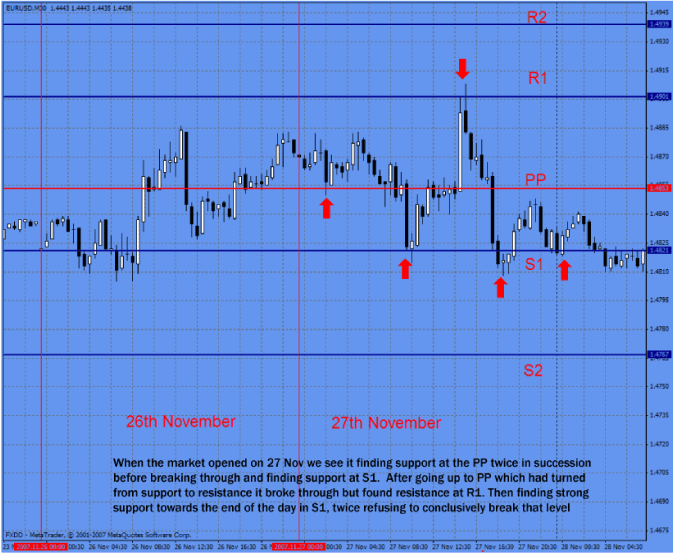# Technical Analysis Part III-Section XI: Pivot Points

Section XI: Pivot Points
In a few words, a pivot point (PP) is a level in which the sentiment of traders and investors changes from bull to bear or vice versa.
Why PP works?
They work simply because many traders and investors (including bank and institutional traders) use and trust them. It is known by every trader that the pivot point is an important measure of strength or weakness of any market.
There are several ways to calculate the pivot point. The method we found to have the most accurate results is calculated by taking the average of the high, low and close of a previous period (or session).
Warning – some pretty boring maths ahead. The good news is that almost all charting platforms will automatically calculate this for you and draw the lines on in whatever pretty colour you like. But as this is the ADVANCED course we think you should have a basic idea of how they are calculated.
Pivot point (PP) = (High + Low + Close) / 3
Take for instance the following EUR/USD information from the previous session:
Open: 1.2386
High: 1.2474
Low: 1.2376
Close: 1.2458
The PP would be,
PP = (1.2474 + 1.2376 + 1.2458) / 3 = 1.2436
So, what does this number tell us? It simply tells us that if the market is trading above 1.2439, Bulls are winning the battle pushing the prices higher. In addition, if the market is trading below this 1.2439 the bears are winning the battle pulling prices lower. In both cases this condition is likely to sustain until the next session.
Since the Forex market is a 24hr market (no close or open from day to day) there has been an ongoing battle deciding at what times we should take the open, close, high and low from each session. From our point of view, the times that produce more accurate predictions is taking the open at 00:00 GMT and the close at 23:59 GMT (obviously the high and low in between those hours).
Besides the calculation of the PP, there are other support and resistance levels that are calculated using the PP as a reference.
Support 1 (S1) = (PP * 2) – H
Resistance 1 (R1) = (PP * 2) - L
Support 2 (S2) = PP – (R1 – S1)
Resistance 2 (R2 ) = R1 + (PP – S1)
Where, H is the High of the previous period
L is the low of the previous period
Continuing with the example above, PP = 1.2436
S1 = (1.2436 * 2) - 1.2474 = 1.2398
R1 = (1.2436 * 2) – 1.2376 = 1.2496
R2 = 1.2496 + (1.2436 – 1.2398) = 1.2338
S2 = 1.2436 – (1.2496 – 1.2398) = 1.2534
These levels are supposed to mark support and resistance levels for the current session.
In the next chart we have calculated the PP and the support and resistance levels for September 5th.
S2 = 1.2616
S1 = 1.2579
PP = 1.2545
R1 = 1.2508
R2 = 1.2474[Chart 1]
Vertical lines separate sessions (4th and 5th of September). As we can see, the market went rapidly below the PP level. From that point on, we should be careful with longs, and start thinking on shorts, because the sentiment of traders and investors is turning to “net short”.
Notice how the PP represents a resistance on early September 5th. Notice also S1 rejected twice the price as it approached the S1 level representing good trading opportunities. Finally, S2 marked a good support, this indicates weakness on current bears, which tells us that the sentiment is not as strong as it was at the beginning of the trading session.
On the example above, the PP was calculated using information of the previous session (previous day). This way we could see possible intraday resistance and support levels. But it can also be calculated using the previous weekly or monthly data to determine such levels. By doing so, we are able to see the sentiment over longer periods of time. In addition, we can see possible levels that might offer support and resistance throughout the week or month. Calculating the weekly or monthly pivot point is mostly used by long term traders, and as we said, it gives us a good idea about the longer term trend.
Got it? Good - there will be questions on this in the quiz later…
As mentioned; all these mapping indicators are available on a point and click basis on almost all charting packages as they are so commonly used (therefore have such intrinsic power). We prefer and choose to use MetaTrader 4 and will go into more detail about that package later.